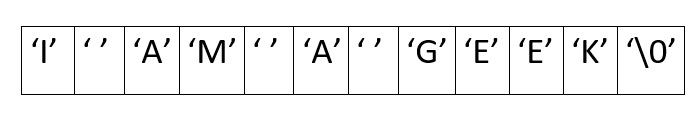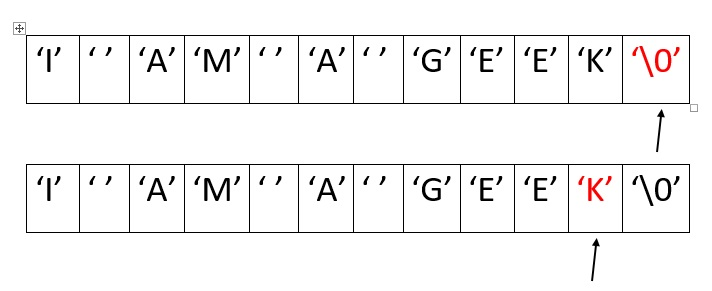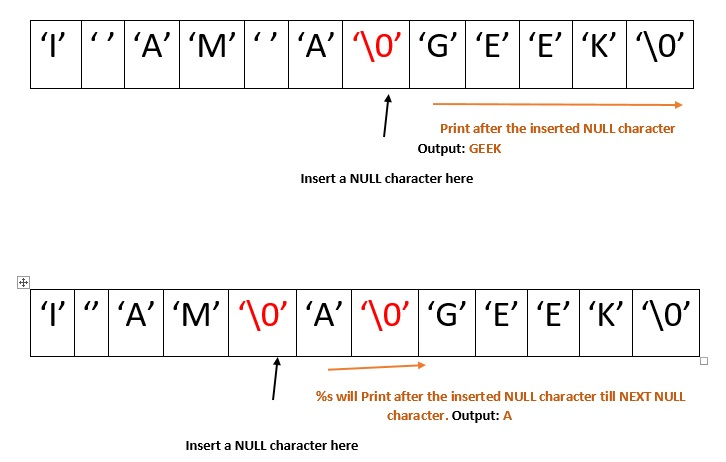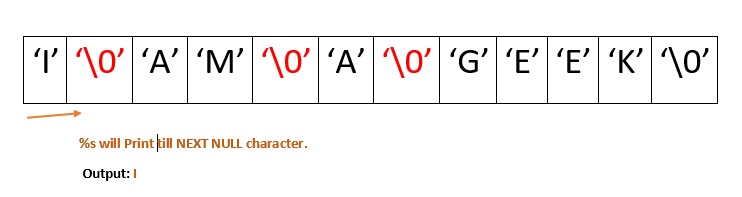Skip to content
Related Articles
Print words of a string in reverse order
• Difficulty Level : Medium
• Last Updated : 26 Mar, 2021

Let there be a string say “I AM A GEEK”. So, the output should be “GEEK A AM I” . This can done in many ways. One of the solutions is given in Reverse words in a string .

Examples:

```Input : I AM A GEEK
Output : GEEK A AM I

Input : GfG IS THE BEST
Output : BEST THE IS GfG```

This can be done in more simpler way by using the property of the “%s format specifier” .
Property: %s will get all the values until it gets NULL i.e. ‘\0’.

Example: char String[] = “I AM A GEEK” is stored as shown in the image below :Approach: Traverse the string from the last character, and move towards the first character. While traversing, if a space character is encountered, put a NULL in that position and print the remaining string just after the NULL character. Repeat this until the loop is over and when the loop ends, print the string, the %s will make the printing of characters until it encounters the first NULL character.

Let us see the approach with the help of diagrams:
step 1: Traverse from the last character until it encounters a space character .Step 2: Put a NULL character at the position of space character and print the string after it.Step 3: At the end, the loop ends when it reaches the first character, so print the remaining characters, it will be printed the first NULL character, hence the first word will be pprinted.## C++

 `// C++ program to print reverse``// of words in a string.``#include` `using` `namespace` `std;` `string wordReverse(string str)``{``    ``int` `i = str.length() - 1;``    ``int` `start, end = i + 1;``    ``string result = ``""``;``    ` `    ``while``(i >= 0)``    ``{``        ``if``(str[i] == ``' '``)``        ``{``            ``start = i + 1;``            ``while``(start != end)``                ``result += str[start++];``            ` `            ``result += ``' '``;``            ` `            ``end = i;``        ``}``        ``i--;``    ``}``    ``start = 0;``    ``while``(start != end)``        ``result += str[start++];``    ` `    ``return` `result;``}` `// Driver code``int` `main()``{``    ``string str = ``"I AM A GEEK"``;``    ` `    ``cout << wordReverse(str);``    ` `    ``return` `0;``}` `// This code is contributed``// by Imam`

## C

 `// C program to print reverse of words in``// a string.``#include ``#include ` `void` `printReverse(``char` `str[])``{``    ``int` `length = ``strlen``(str);` `    ``// Traverse string from end``    ``int` `i;``    ``for` `(i = length - 1; i >= 0; i--) {``        ``if` `(str[i] == ``' '``) {` `            ``// putting the NULL character at the``            ``// position of space characters for``            ``// next iteration.        ``            ``str[i] = ``'\0'``;` `            ``// Start from next charatcer    ``            ``printf``(``"%s "``, &(str[i]) + 1);``        ``}``    ``}` `    ``// printing the last word``    ``printf``(``"%s"``, str);``}` `// Driver code``int` `main()``{``    ``char` `str[] = ``"I AM A GEEK"``;``    ``printReverse(str);``    ``return` `0;``}`

## Java

 `// Java program to print reverse``// of words in a string.``import` `java.io.*;``import` `java.util.*;``import` `java.lang.*;` `class` `GFG``{``static` `String wordReverse(String str)``{``    ``int` `i = str.length() - ``1``;``    ``int` `start, end = i + ``1``;``    ``String result = ``""``;``    ` `    ``while``(i >= ``0``)``    ``{``        ``if``(str.charAt(i) == ``' '``)``        ``{``            ``start = i + ``1``;``            ``while``(start != end)``                ``result += str.charAt(start++);``            ` `            ``result += ``' '``;``            ` `            ``end = i;``        ``}``        ``i--;``    ``}``    ` `    ``start = ``0``;``    ``while``(start != end)``        ``result += str.charAt(start++);``    ` `    ``return` `result;``}` `// Driver code``public` `static` `void` `main(String[] args)``{``    ``String str = ``"I AM A GEEK"``;``    ` `    ``System.out.print(wordReverse(str));``}``}` `// This code is contributed``// by Akanksha Rai(Abby_akku)`

## Python3

 `# Python3 program to print reverse ``# of words in a string.` `def` `wordReverse(``str``):``    ``i ``=` `len``(``str``)``-``1``    ``start ``=` `end ``=` `i``+``1``    ``result ``=` `''` `    ``while` `i>``=``0``:``        ``if` `str``[i] ``=``=` `' '``:``            ``start ``=` `i``+``1``            ``while` `start!``=` `end:``                ``result ``+``=` `str``[start]``                ``start``+``=``1``            ``result``+``=``' '``            ``end ``=` `i``        ``i``-``=``1``    ``start ``=` `0``    ``while` `start!``=``end:``        ``result``+``=``str``[start]``        ``start``+``=``1``    ``return` `result` `# Driver Code``str` `=` `'I AM A GEEK'``print``(wordReverse(``str``))` `# This code is contributed ``# by SamyuktaSHegde`

## C#

 `// C# program to print reverse``// of words in a string.``using` `System;``class` `GFG``{``static` `String wordReverse(String str)``{``    ``int` `i = str.Length - 1;``    ``int` `start, end = i + 1;``    ``String result = ``""``;``    ` `    ``while``(i >= 0)``    ``{``        ``if``(str[i] == ``' '``)``        ``{``            ``start = i + 1;``            ``while``(start != end)``                ``result += str[start++];``            ` `            ``result += ``' '``;``            ` `            ``end = i;``        ``}``        ``i--;``    ``}``    ` `    ``start = 0;``    ``while``(start != end)``        ``result += str[start++];``    ` `    ``return` `result;``}` `// Driver code``public` `static` `void` `Main()``{``    ``String str = ``"I AM A GEEK"``;``    ` `    ``Console.Write(wordReverse(str));``}``}` `// This code is contributed``// by Akanksha Rai(Abby_akku)`

## PHP

 `= 0)``    ``{``        ``if``(``\$str``[``\$i``] == ``' '``)``        ``{``            ``\$start` `= ``\$i` `+ 1;``            ``while``(``\$start` `!= ``\$end``)``                ``\$result` `= ``\$result` `. ``\$str``[``\$start``++];``            ` `            ``\$result` `= ``\$result` `. ``' '``;``            ` `            ``\$end` `= ``\$i``;``        ``}``        ``\$i``--;``    ``}``    ``\$start` `= 0;``    ``while``(``\$start` `!= ``\$end``)``        ``\$result` `= ``\$result` `. ``\$str``[``\$start``++];``    ` `    ``return` `\$result``;``}` `// Driver code``\$str` `= ``"I AM A GEEK"``;``echo` `wordReverse(``\$str``);` `// This code is contributed by ita_c``?>`

## Javascript

 ``

Output:

`GEEK A AM I`

Time Complexity: O(len(str))

Auxiliary Space: O(len(str))

This article is contributed by MAZHAR IMAM KHAN. If you like GeeksforGeeks and would like to contribute, you can also write an article using contribute.geeksforgeeks.org or mail your article to contribute@geeksforgeeks.org. See your article appearing on the GeeksforGeeks main page and help other Geeks.
Please write comments if you find anything incorrect, or you want to share more information about the topic discussed above.

Attention reader! Don’t stop learning now. Get hold of all the important DSA concepts with the DSA Self Paced Course at a student-friendly price and become industry ready.  To complete your preparation from learning a language to DS Algo and many more,  please refer Complete Interview Preparation Course.

In case you wish to attend live classes with industry experts, please refer DSA Live Classes

My Personal Notes arrow_drop_up